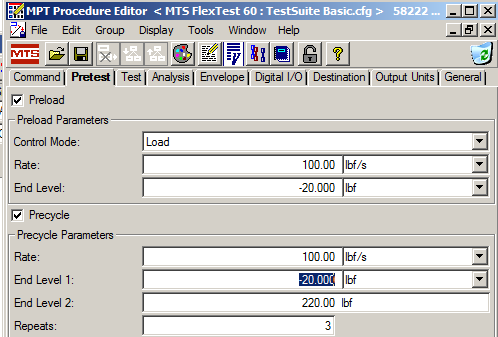You do not have permission to access this content.
To request access, please submit a support request here.

# How do you get a whole number of precycles to run in the MPT Elastomer Static Deflection Pretest Precycle activity?

QUESTION

How do you get a whole number of precycles to run in the MPT Elastomer Static Deflection Pretest Precycle activity?

You may encounter the problem of not being able to get a whole number of cycles in the Precycle portion of the MPT Static Deflection Activity (Figure 1)?  For example, if you choose Repeats: 3 times you may end up with 2.5 cycles instead of 3 (Figure 2).Figure 1 – Results of Static Deflection PrecycleFigure 2 – Static Deflection Precycle Configuration

In the precycle, 1 cycle is considered to be a ramp to end-level 1 and then a ramp to end-level 2.  It will always stop at end-level 2.  Now if you enable the preload step before the precycle it will first ramp to the preload level, do the precycle (ending at end-level 2), then ramp back to the preload before moving onto the test cycles. Sequence as follows (Figure 3).

1. Preload ramps to -20 lbf.
2. Cycle 1 End level 1 is already at -20 lbf so only goes to 2,220 lbf to complete cycle 1.
3. Cycle 2 is ramp to end level 1 -20 lbf then ramp to end level 2 2,200 lbf.
4. Cycle 3 is ramp to end level 1 -20 lbf to end level 2 2,200 lbf.
5.  Because we had a preload of -20 lbf it finishes by going back to the pre-load -20 lbf.Figure 3 – Preload and Precycle Sequence

Setup below (Figure 4).Figure 4 – Pretest Parameter Setup

Results in desired outcome of 3 full cycles (Figure 5).Figure 5 – Results of Preload and Precycle

K
Keary is the author of this solution article.

Did you find it helpful? Yes No

Send feedback